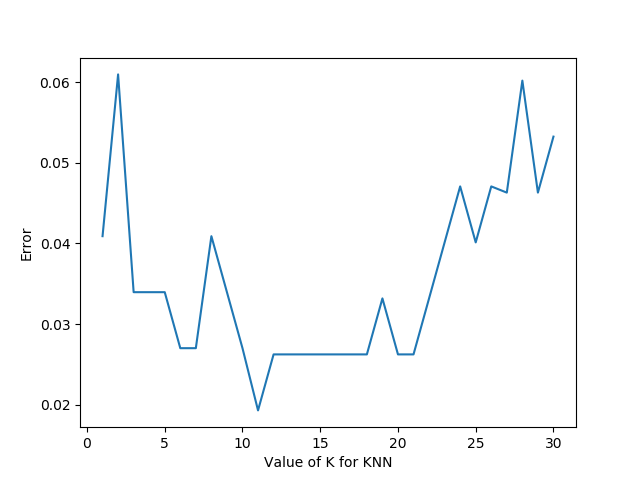# 一.Skelarn KNN参数概述

def KNeighborsClassifier(n_neighbors = 5,
weights='uniform',
algorithm = '',
leaf_size = '30',
p = 2,
metric = 'minkowski',
metric_params = None,
n_jobs = None
)

- n_neighbors：这个值就是指 KNN 中的 “K”了。前面说到过，通过调整 K 值，算法会有不同的效果。
- weights（权重）：最普遍的 KNN 算法无论距离如何，权重都一样，但有时候我们想搞点特殊化，比如距离更近的点让它更加重要。这时候就需要 weight 这个参数了，这个参数有三个可选参数的值，决定了如何分配权重。参数选项如下：
• 'uniform'：不管远近权重都一样，就是最普通的 KNN 算法的形式。
• 'distance'：权重和距离成反比，距离预测目标越近具有越高的权重。
• 自定义函数：自定义一个函数，根据输入的坐标值返回对应的权重，达到自定义权重的目的。
- algorithm：在 sklearn 中，要构建 KNN 模型有三种构建方式，1. 暴力法，就是直接计算距离存储比较的那种放松。2. 使用 kd 树构建 KNN 模型 3. 使用球树构建。 其中暴力法适合数据较小的方式，否则效率会比较低。如果数据量比较大一般会选择用 KD 树构建 KNN 模型，而当 KD 树也比较慢的时候，则可以试试球树来构建 KNN。参数选项如下：
• 'brute' ：蛮力实现
• 'kd_tree'：KD 树实现 KNN
• 'ball_tree'：球树实现 KNN
• 'auto'： 默认参数，自动选择合适的方法构建模型

- leaf_size：如果是选择蛮力实现，那么这个值是可以忽略的，当使用KD树或球树，它就是是停止建子树的叶子节点数量的阈值。默认30，但如果数据量增多这个参数需要增大，否则速度过慢不说，还容易过拟合。
- p：和metric结合使用的，当metric参数是"minkowski"的时候，p=1为曼哈顿距离， p=2为欧式距离。默认为p=2。
- metric：指定距离度量方法，一般都是使用欧式距离。
• 'euclidean' ：欧式距离
• 'manhattan'：曼哈顿距离
• 'chebyshev'：切比雪夫距离
• 'minkowski'： 闵可夫斯基距离，默认参数
- n_jobs：指定多少个CPU进行运算，默认是-1，也就是全部都算。



# 二. KNN代码实例

KNN算法算是机器学习里面最简单的算法之一了，我们来sklearn官方给出的例子，来看看KNN应该怎样使用吧：from sklearn.datasets import load_iris
from sklearn.model_selection  import cross_val_score
import matplotlib.pyplot as plt
from sklearn.neighbors import KNeighborsClassifier

#读取鸢尾花数据集
x = iris.data
y = iris.target
k_range = range(1, 31)
k_error = []
#循环，取k=1到k=31，查看误差效果
for k in k_range:
knn = KNeighborsClassifier(n_neighbors=k)
#cv参数决定数据集划分比例，这里是按照5:1划分训练集和测试集
scores = cross_val_score(knn, x, y, cv=6, scoring='accuracy')
k_error.append(1 - scores.mean())

#画图，x轴为k值，y值为误差值
plt.plot(k_range, k_error)
plt.xlabel('Value of K for KNN')
plt.ylabel('Error')
plt.show()import matplotlib.pyplot as plt
from numpy import *
from matplotlib.colors import ListedColormap
from sklearn import neighbors, datasets

n_neighbors = 11

# 导入一些要玩的数据
x = iris.data[:, :2]  # 我们只采用前两个feature,方便画图在二维平面显示
y = iris.target

h = .02  # 网格中的步长

# 创建彩色的图
cmap_light = ListedColormap(['#FFAAAA', '#AAFFAA', '#AAAAFF'])
cmap_bold = ListedColormap(['#FF0000', '#00FF00', '#0000FF'])

#weights是KNN模型中的一个参数，上述参数介绍中有介绍，这里绘制两种权重参数下KNN的效果图
for weights in ['uniform', 'distance']:
# 创建了一个knn分类器的实例，并拟合数据。
clf = neighbors.KNeighborsClassifier(n_neighbors, weights=weights)
clf.fit(x, y)

# 绘制决策边界。为此，我们将为每个分配一个颜色
# 来绘制网格中的点 [x_min, x_max]x[y_min, y_max].
x_min, x_max = x[:, 0].min() - 1, x[:, 0].max() + 1
y_min, y_max = x[:, 1].min() - 1, x[:, 1].max() + 1
xx, yy = np.meshgrid(np.arange(x_min, x_max, h),
np.arange(y_min, y_max, h))
Z = clf.predict(np.c_[xx.ravel(), yy.ravel()])

# 将结果放入一个彩色图中
Z = Z.reshape(xx.shape)
plt.figure()
plt.pcolormesh(xx, yy, Z, cmap=cmap_light)

# 绘制训练点
plt.scatter(x[:, 0], x[:, 1], c=y, cmap=cmap_bold)
plt.xlim(xx.min(), xx.max())
plt.ylim(yy.min(), yy.max())
plt.title("3-Class classification (k = %i, weights = '%s')"
% (n_neighbors, weights))

plt.show()


## KNN和Kmeans

1. K值都是重点
2. 都需要计算平面中点的距离

Knn和Kmeans的核心都是通过计算空间中点的距离来实现目的，只是他们的目的是不同的。KNN的最终目的是分类，而Kmeans的目的是给所有距离相近的点分配一个类别，也就是聚类。

posted @ 2019-04-10 18:18  zzzzMing  阅读(32948)  评论(1编辑  收藏  举报